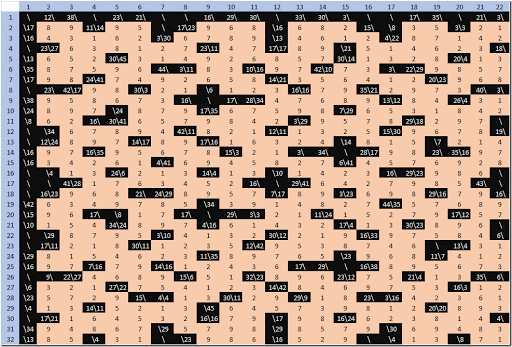## Saturday, February 18, 2017

### Solving Kakuro puzzles as a MIP

The Kakuro puzzle is another logical puzzle similar to Sudoku, KenKen and Takuzu. A small example from (1) is:

The rules are as follows:

1. Each (white) cell must be filled with a value $$1,..,9$$.
2. Each (white) cell belongs to a horizontal and/or a vertical block of contiguous cells.
3. The values of the cells in each block add up to a given constant. These constants are to the left (or top) of the block. A notation a\b is used: a is the sum for the block below and b is the sum for the block to the right.
4. The values in each block must be unique (i.e. no duplicates).
##### Model

The actual model is rather simple. Similar to (2) and (3) I define a binary decision variable:

 $x_{i,j,k} = \begin{cases}1 & \text{if cell (i,j) has the value k} \\ 0& \text{otherwise}\end{cases}$

where $$k=\{1,..,9\}$$. Of course, we can select only one value in a cell, i.e.:

 $\sum_k x_{i,j,k} = 1 \>\>\forall i,j|IJ(i,j)$

where $$IJ(i,j)$$ is the set of white cells we need to fill in. To make the model simpler we also define an integer variable $$v_{i,j}$$ with the actual value:

 $v_{i,j} = \sum_k k\cdot x_{i,j,k}$

The values in each block need to add up the given constant. So:

 $\sum_{i,j|B(b,i,j)} v_{i,j} = C_b \>\>\forall b$

where the set $$B(b,i,j)$$ is the mapping between block number and cells $$(i,j)$$. The uniqueness constraint is not very difficult at all:

 $\sum_{i,j|B(b,i,j)} x_{i,j,k} \le 1 \>\>\forall b,k$

Note that (1) uses a different numbering scheme for the cells, but otherwise the models are very similar. My version of the model is smaller in terms of nonzero elements in the matrix, due to my use of intermediate variables $$v_{i,j}$$.

##### Data entry

A compact way to enter the data for the example problem in GAMS is:

 parameter  block(b,i,j) /    block1.r1.(c1*c2)   16  ,   block13.(r1*r3).c1  23    block2.r1.(c5*c7)   24  ,   block14.(r6*r7).c1  11    block3.r2.(c1*c2)   17  ,   block15.(r1*r4).c2  30    block4.r2.(c4*c7)   29  ,   block16.(r6*r7).c2  10    block5.r3.(c1*c5)   35  ,   block17.(r3*r7).c3  15    block6.r4.(c2*c3)    7  ,   block18.(r2*r3).c4  17    block7.r4.(c5*c6)    8  ,   block19.(r5*r6).c4   7    block8.r5.(c3*c7)   16  ,   block20.(r1*r5).c5  27    block9.r6.(c1*c4)   21  ,   block21.(r1*r2).c6  12    block10.r6.(c6*c7)   5  ,   block22.(r4*r7).c6  12    block11.r7.(c1*c3)   6  ,   block23.(r1*r2).c7  16    block12.r7.(c6*c7)   3  ,   block24.(r5*r7).c7   7  /

The sets $$IJ(i,j)$$ and parameter $$C_b$$ can be easily extracted from this.

##### Results

After adding a dummy objective, we can solve the model as a MIP (Mixed Integer Programming) Model. The output looks like:

 ----     75 VARIABLE v.L              c1          c2          c3          c4          c5          c6          c7 r1           9           7                                   8           7           9r2           8           9                       8           9           5           7r3           6           8           5           9           7r4                       6           1                       2           6r5                                   4           6           1           3           2r6           8           9           3           1                       1           4r7           3           1           2                                   2           1

It is noted that although I used the declaration $$x_{i,j}$$, only the subset of cells that we actually need to fill in is used in the equations. GAMS will only actually generate the variables that appear in the equations, so all the “black” cells are not part of the model.

Cplex can solve the model in zero iterations and zero nodes: it solves the model completely in the presolve.

Interestingly in (1) somewhat disappointing performance results are reported. They use CBC and a solver called eplex (never heard of that one). I checked the above small instance with CBC and it can solve it in zero nodes, just like Cplex.

##### A large, difficult example

This example is taken from (4):

For this problem Cplex has to do a little bit of work. The log looks like:

 ---   3,665 rows  4,921 columns  19,189 non-zeroes---   4,920 discrete-columns . . . Dual simplex solved model. Root relaxation solution time = 0.44 sec. (102.53 ticks)         Nodes                                         Cuts/   Node  Left     Objective  IInf  Best Integer    Best Bound    ItCnt     Gap       0     0        0.0000   325                      0.0000     1727               0     0        0.0000   188                    Cuts: 39     2562               0     0        0.0000   233                    Cuts: 49     3018               0     0        0.0000   227                    Cuts: 18     3403               0     0        0.0000   147                    Cuts: 64     4037         *     0+    0                            0.0000        0.0000             0.00%Found incumbent of value 0.000000 after 4.42 sec. (1071.33 ticks)      0     0        cutoff              0.0000        0.0000     4423    0.00%Elapsed time = 4.44 sec. (1072.36 ticks, tree = 0.01 MB, solutions = 1). . . Proven optimal solution. MIP Solution:            0.000000    (4423 iterations, 0 nodes)

To be complete, CBC takes a little more time:

 Search completed - best objective 0, took 55117 iterations and 140 nodes (42.00 seconds)Strong branching done 1748 times (104829 iterations), fathomed 9 nodes and fixed 122 variablesMaximum depth 18, 0 variables fixed on reduced cost

This model does not solve completely in the presolve. However the computation times look quite good to me, especially when compared to what is reported in (1).

The solution looks like:##### Proving uniqueness

We can prove the uniqueness of this solution by adding the constraint:

 $\sum_{i,j,k|IJ(i,j)} x^*_{i,j,k} x_{i,j,k} \le |IJ| –1$

where $$x^*$$ is the previously found optimal solution, and $$|IJ|$$ is the cardinality of the set $$IJ(i,j)$$ (i.e. the number of “white” cells). Note that $$\sum x^*_{i,j,k}=\sum x_{i,j,k} = |IJ|$$. With this constraint we should either find a different solution (so the solution was not unique), or the model should be declared “infeasible”.

##### References
1. Helmut Simonis, Kakuro as a Constraint Problem, Cork Constraint Computation Centre (4C), University College Cork, 2008.
2. MIP Modeling: From Sukodu to Kenken via Logarithms, http://yetanothermathprogrammingconsultant.blogspot.com/2016/10/mip-modeling-from-sudoku-to-kenken.html
3. Solving Takuzu puzzles as a MIP using xor, http://yetanothermathprogrammingconsultant.blogspot.com/2017/01/solving-takuzu-puzzles-as-mip-using-xor.html
4. http://pagesperso.g-scop.grenoble-inp.fr/~cambazah/page1/page7/assets/data_generated_hard.ecl has some larger data sets.
5. http://www.gecode.org/doc-latest/MPG.pdf, Chapter 21 is about solving Kakuro using Gecode, a constraint programming solver.
6. José Barahona da Fonseca, A novel linear MILP model to solve Kakuro puzzles, 2012. This has a (partial) GAMS model (stylistically I would write things differently; the model can be written more cleanly).